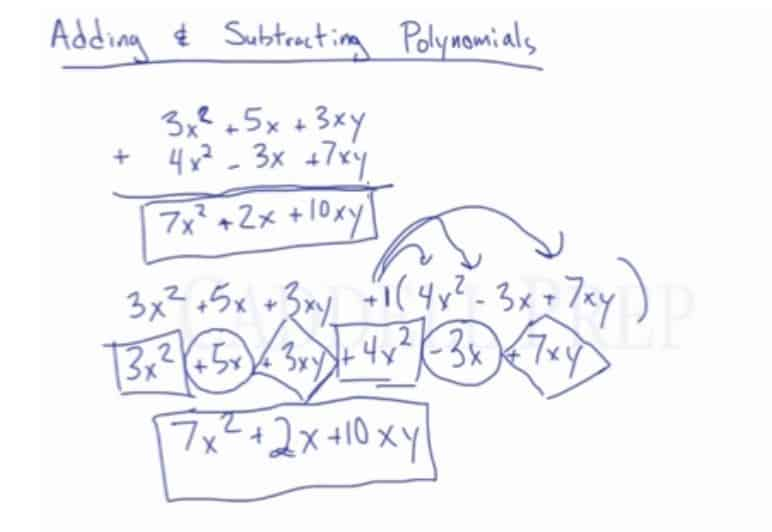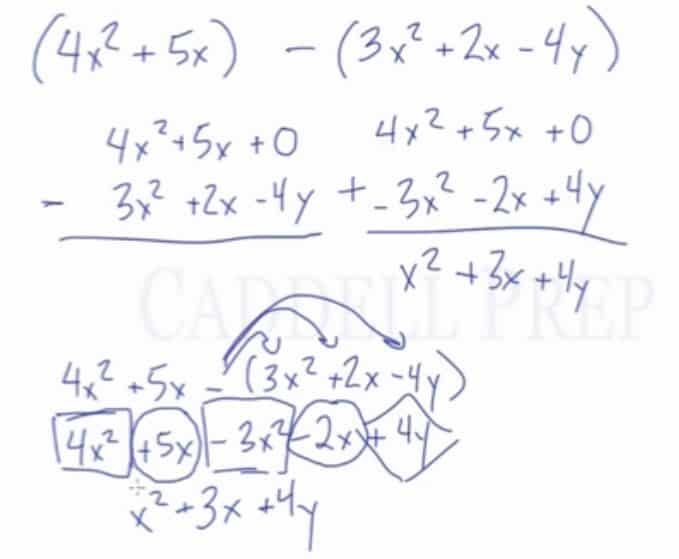In this video, learn how to add & subtract polynomials by combining like terms. After you finish this lesson, view all of our Pre-Algebra lessons and practice problems.

In this video, we are going to look at adding and subtracting polynomials.
For example:
To simplify the expression:$(3x^2+5x+3xy)+(4x^2-3x+7xy)$
combine the like terms to get the final answer of$7x^2+2x+10xy$
To simplify the expression:$(3x^2+5x+3xy)-(4x^2-3x+7xy)$
we can either keep-change-change or distribute the negative to get$(3x^2+5x+3xy)+(-4x^2+3x-7xy)$
Then, combine like terms to get the final answer of$-x^2+8x-4xy$
Sometimes, every term may not line up, such as in the expression$(4x^2+5x)-(3x^2+2x-4y)$
Solve as you would normally by either keep-change-change or distributing the negative, and then combining like terms to get a final answer of$x^2+3x+4y$

## Examples of Adding and Subtracting Polynomials

### Example 1$(12x^2+ 17y - 4) + (9x^2- 13y + 3)$

Combine the like terms

Now, we have$21x^2+4y-1$

### Example 2$(5x^2- 2x - 1) - (3x^2- 5x + 7)$

First, distribute the negative sign to the terms inside the second parenthesis$5x^2- 2x - 1 - 3x^2+5x - 7$

Then, combine the like terms

Now, we have$2x^2+3x-8$

## Video-Lesson Transcript

Now, let’s discuss how to add and subtract polynomials.

For example, we have$3x^2 + 5x + 3xy$$+ 4x^2 - 3x + 7xy$

It’s helpful to align the like terms so its easier to combine them.

Now, we combine the like terms$7x^2 + 2x + 10xy$

Or we can write the two polynomials on a straight line like this:$3x^2 + 5x + 3xy + (4x^2 - 3x + 7xy)$

Now, we distribute the$+$ sign.

It’s like we have$+1$ at the beginning of the polynomial inside the parenthesis.

So we’ll have$3x^2 + 5x + 3xy + 4x^2 - 3x + 7xy$

Then, look for the like terms and combine them.$7x^2 + 2x + 10xy$

We got the same answer as the first.Let’s look at an example which involves subtraction.$3x^2 + 5x + 3xy$$- 4x^2 - 3x + 7xy$

Since we’re subtracting polynomials, we should do keep-change-change.

Let’s keep the top the same.$3x^2 + 5x + 3xy$

Lastly, we change the signs of each term.$-4x^2 + 3x - 7xy$

Now, it’s time for us to combine the like terms$3x^2 + 5x + 3xy$$+ (-4x^2) + 3x - 7xy$$-x^2 + 8x - 4xy$

Now, let’s do this on a straight line.$3x^2 + 5x + 3xy - (4x^2 - 3x + 7xy)$

Let’s distribute the negative sign into the polynomial in the parenthesis. Just like there’s a$-1$ at the beginning.

So, we’ll have$3x^2 + 5x + 3xy - 4x^2 + 3x - 7xy$

Then, combine the like terms. We now have$-x^2 + 8x - 4xy$

Let’s look at a pretty difficult problem here$(4x^2 + 5x) - (3x^2 + 2x - 4y)$

Let’s solve this using the two-row format.$4x^2 + 5x$$- 3x^2 + 2x - 4y$

Since there’s nothing above the$4y$, we can put in$0$ as a place holder.

Then, let’s do keep-change-change since we have subtraction.

It will look like this now$4x^2 + 5x + 0$$+ (-3x^2) - 2x + 4y$

After combining the like terms, the answer is$x^2 + 3x + 4y$

Now, let’s solve in a horizontal line format$4x^2 + 5x - (3x^2 + 2x - 4y)$$4x^2 + 5x - 3x^2 - 2x + 4y$$x^2 + 3x + 4y$